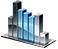# Loops

Loop statements are used to repeatedly execute certain code provided that the certain condition is true.

## While statements

If the condition is true (has the value true ), then the block of operators in the curly brackets `{}` is executed and after that the verification of conditions repeats. If the condition is false , the execution of the loop stops.

Example:

` int i=1; while(i<2000) { i *=2; System.Print("i="+i); } `

## Do-while statement

This statement is used for creating a loop with a postcondition. It differs from a simple operator ` while ` by the loop body that is executed at least once. The condition is verified at the end of the loop, not at its beginning.

Example:

` int a=0; do { a++; System.Print("a="+a); } while(a<5); `

## For statement

The main difference between the loop ` for `and the loop ` while ` is in the parameters of the loop. In the loop ` for `, you can set a variable that will work as a counter of loop iterations, the initial value can be set by this variable, and it is also allowed to determine the condition of the loop and change the value of this variable. In general, the loop ` for ` has the following syntax:

` for(initial_value; execution_condition; increment) { operators; } `

Expressions initial_value , execution_condition and increment may be missing, but the semicolons `; ` are compulsory.

Example:

` for (int i = 1; i < 10; i++) { System.Print("i="+i); } `